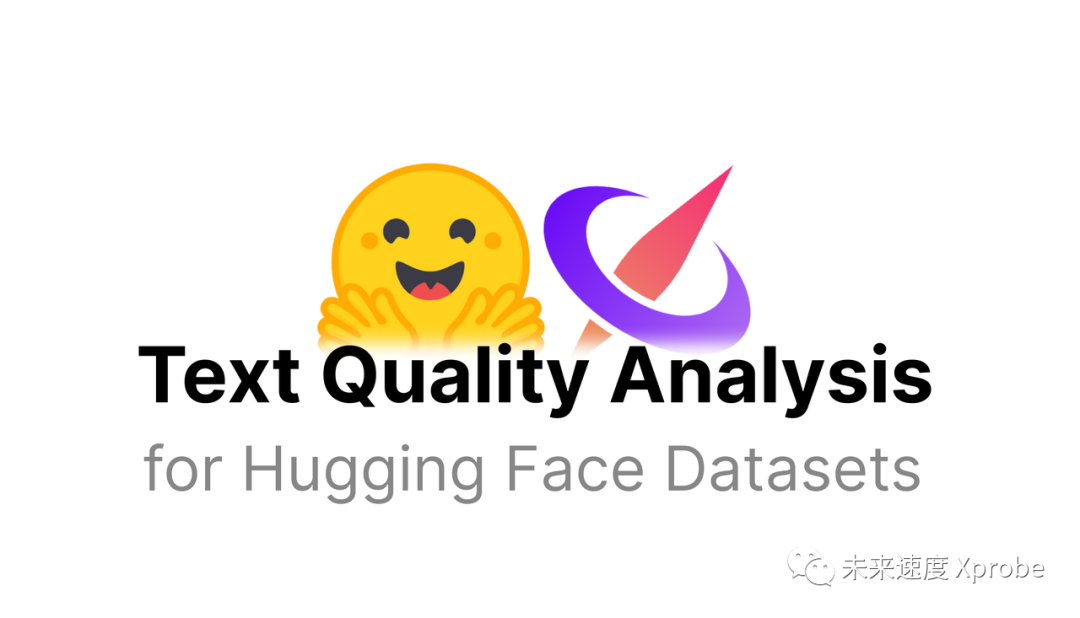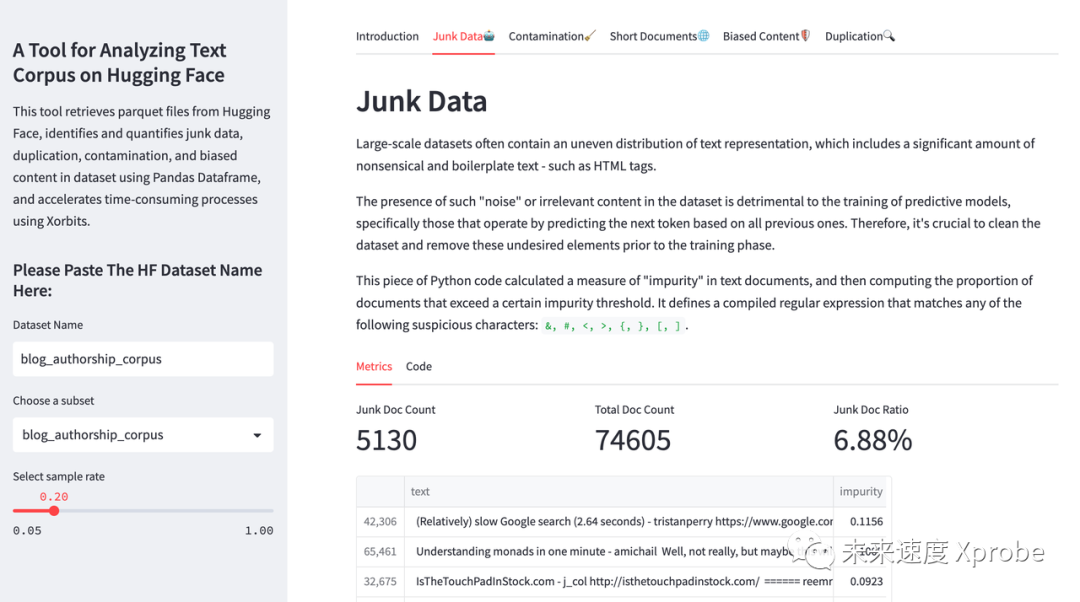# 社区供稿 | Hugging Face Datasets 文本质量分析

07/13 18:00## 数据集质量的重要性

HuggingFace-Datasets-Text-Quality-Analysis这个项目的目标就是让人们评估Hugging Face上文本类型数据集的质量。这个工具可以从Hugging Face获取parquet文件，然后识别出数据集中的垃圾数据、重复数据、污染数据、偏见内容等质量问题。## 如何使用？

$conda create -n datasets-quality python=3.9$ conda activate datasets-quality

$cd HuggingFace-Datasets-Text-Quality-Analysis$ pip install -r requirements.txt最后，运行Streamlit应用：python -m streamlit run app.py

### 注意事项

https://github.com/xorbitsai/xorbits

### 识别低质量内容

import reRE_SUSPICIOUS = re.compile(r'[\u0026#\u003c\u003e{}\$\$\\\\]')def impurity(text, min_len=10):    """returns the share of suspicious characters in a text"""    if text == None or len(text) < min_len:        return 0    else:        return len(RE_SUSPICIOUS.findall(text))/len(text)df['impurity'] = df['text'].apply(impurity, min_len=10)total_num_docs = len(df)impurity_num_docs = len(df[df['impurity'] > 0.01])impurity_ratio = impurity_num_docs / total_num_docs

### 识别毒性内容

The Pile使用了一个叫Spam Scanner的工具对有害内容进行分类。Spam Scanner是一款用于限制垃圾邮件，防止网络钓鱼和对用户的攻击的API工具，它内置了一个毒性检测的分类器；在tfjs-models中，也包含了一个检测毒性内容的分类器。

with open('./List-of-Dirty-Naughty-Obscene-and-Otherwise-Bad-Words', 'r') as f:    lines = f.readlines()    banned_words = [line.rstrip('\n') for line in lines]    df['banned_words_in_text'] = df['text'].apply(lambda text: [word for word in banned_words if word in text.lower().split()])     total_num_docs = len(df)    df['matches'] = df['banned_words_in_text'].apply(lambda words: len(words) > 0)    biased_num_docs = df['matches'].sum()    biased_content_ratio = biased_num_docs / total_num_docs 

### 检测重复文档

from datasketch import MinHashLSH, MinHashdf = train_datasetlsh = MinHashLSH(threshold=0.85, num_perm=128)for i, text in enumerate(df['text']):    minhash = MinHash(num_perm=128)    for word in text.split():        minhash.update(word.encode('utf-8'))    lsh.insert(str(i), minhash)unique_documents = set()for i, text in enumerate(df['text']):    query_minhash = MinHash(num_perm=128)    for word in text.split():        query_minhash.update(word.encode('utf-8'))    results = lsh.query(query_minhash)    unique_documents.add(results)total_unique_documents = len(unique_documents)total_documents = len(df)duplication_ratio = (total_documents - total_unique_documents) / total_documentsduplication_ratio

### 识别测试集污染数据

OpenAI定义了一个测试文档，如果它与任何训练文档存在任何N-gram的重叠，那么它就被认为是被污染的。（他们根据数据集使用了在8到13之间的一系列N值。）在构建WebText数据集时，OpenAI的研究人员通过从训练集中消除所有的维基百科内容来去污染数据。这是必要的，因为维基百科的数据在他们的基准测试数据集中被大量使用。

from nltk import ngramsfrom datasketch import MinHash, MinHashLSHdef process_data(df):    minhashes = {}    for idx, text in enumerate(df['text']):        minhash = MinHash(num_perm=128)        for d in ngrams(text, 13):            s = "".join(d).encode('utf-8')            minhash.update(s)        minhashes[idx] = minhash    return minhashestrain_minhashes = process_data(train_dataset)test_minhashes = process_data(test_dataset)lsh = MinHashLSH(threshold=0.8, num_perm=128)for idx, minhash in train_minhashes.items():    lsh.insert(idx, minhash)duplicates_count = 0for idx, minhash in test_minhashes.items():    result = lsh.query(minhash)    if len(result) > 0:        duplicates_count += 1contamination_ratio = duplicates_count / len(test_dataset)contamination_ratio

### 识别过短的内容

from transformers import BertTokenizertokenizer = BertTokenizer.from_pretrained('bert-base-uncased')df = train_dataset# Create a new column with the number of tokens for each textdf['text_length'] = df['text'].apply(lambda text: len(tokenizer.tokenize(text)))total_num_docs = len(df)too_short_docs = len(df[df['text_length'] < 100])too_short_doc_ratio = too_short_docs / total_num_docstoo_short_doc_ratio

### 最后0 评论
0 收藏
0